﻿ Hydrodynamic Characteristic of the Marine Propeller in the Oblique Flow with Various Current Angle by CFD SolverPublications are Open
Access in this journal
Article Versions
Export Article
• Normal Style
• MLA Style
• APA Style
• Chicago Style
Research Article
Open Access Peer-reviewed

### Hydrodynamic Characteristic of the Marine Propeller in the Oblique Flow with Various Current Angle by CFD Solver

Alireza Abbasi, Hassan Ghassemi , Manouchehr Fadavie
American Journal of Marine Science. 2018, 6(1), 25-29. DOI: 10.12691/marine-6-1-3
Received June 29, 2018; Revised August 02, 2018; Accepted August 09, 2018

### Abstract

The purpose of this study is to obtain the hydrodynamic characteristic of propeller in the oblique flow with various current angle (means oblique flow). The Reynolds-averaged Navier-Stokes (RANS) equations solver of the StarCCM+ software is employed with a realizable k-ε turbulent model. In the current study, the B-Series propeller (B:4-70) is used because of its use in commercial vessels. The simulation is conducted in two parts: at first, examination of propeller behavior in direct flow conditions and comparison with experimental conditions and in the next step analysis of the propeller in the oblique flow with angles of 10, 20, 30 and 40 degrees. The results are shown that the thrust and torque coefficients (KTx and KQx) are reduced by increasing the angle of flow and increasing the advance coefficient.

### 1. Introduction

In recent years, computational fluid dynamics models have shown that they are very suitable method for analyzing the hydrodynamic behavior of marine propellers. Although there are holes in complex simulations, such as cavitation and two-phase currents, there are good numerical researches in the fields as well. Conventional propulsive systems such as propellers are mainly designed for direct flow conditions. When marine propellers are in a non-direct current such as maneuvering or stopping and turning, their hydrodynamic performance varies considerably. Marine vessels experience different performance during their lifetime. One of the most important issues in the field of hydrodynamics is the flow analysis around the marine vessels during maneuvering. During the maneuver the vessel, the propeller is exposed to the oblique flow and its function changes. These unstable hydrodynamic loads, from the view of structurally and functionally, can be important and even destructive. Nowadays, hydrodynamic analysis of the flow during the maneuvers of floats has become a major challenge. Hence, due to the existing needs, researchers are seeking to gain more efficiency from the propulsion system in conditions other than their design conditions, so they use numerical and experimental methods.

Experiments and numerical simulations have provided valuable information on the hydrodynamic performance of propellers in oblique flow conditions. Shamsi et al carried out the hydrodynamic performance of the propeller at oblique flow and off-design conditions 1, 2, 3. Viviani et al. made empirical research on the behavior of propellers during a maneuver on a circular path 4. These experiments were used to estimate the asymmetric distribution of loads applied to the propulsion system. The results showed that the propeller thrust significantly increased in the oblique flow mode. Chang et al. studied the types of performance of the propeller during the stop 5. In the case of a propeller in cross-flow mode, creates a force in the opposite direction of the ship and produces a yaw moment. Experimental and numerical studies of Atsavapranee et al. 6 were one of the work sdone on the impact of the propeller on ship maneuvers. They performed numerical simulations and experiments on the DDG51 destroyer model. Their research showed during maneuvering the propeller produced a transverse flow which would causes hydrodynamic loads to be carried on the vessel surface. Dubbioso et al. 7, 8 applied the RANS Code and have studied on the propeller performance at two different loading modes and with various oblige angles. Shuai Sun et al. used a numerical method to analysis and computing the propeller forces in the propeller-rudder-hull system in oblique flow conditions. The results indicated that the coefficients of the hull resistance caused by lateral forces and yaw moment decrease with decreasing drift angle 9. Numerical prediction analysis of propeller bearing force for full-scale hull-propeller-rudder system carried out by Wang et al. 10. Nowruzi et al 11 performed the predicting of 2D and 3D submerged hydrofoils using CFD and ANNs. Using computational fluid dynamic and artificial neural networks to predict the performance and cavitation volume of a propeller under different geometrical and physical characteristics carried out by Shora et al 12. Recently, Najaf et al presented the performance prediction of hydrofoil-supported catamarans using experiment and ANNs 13.

This study aimed to predict propeller hydrodynamic characteristics of commercial vessels in terms of oblique flow conditions at angles of 10, 20, 30 and 40 degrees using the StarCCM+ software. The propeller chosen for this study is the B-Series of 4-bladed (Z=4) and expanded area ratio (EAR) 0.70, namely B:4-70.

### 2. Computational Method

2.1. Mathematical Formulation

According to the Reynolds number, the flow pattern is turbulent therefore, should be used a suitable turbulence model to exert turbulence effect on flow for this reason K-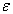turbulence model used in this study. The flow generated by solid body in the fluid is modeled with the RANS equations. The governing equations of fluid flow include the continuity and Navier-Stokes equations. By applying Reynolds averaging, RANS equations will be obtained: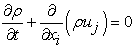(1)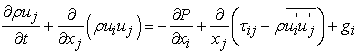(2)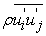represents Reynolds stresses. Based on turbulent viscosity theory that provides relationship between Reynolds stress terms and velocity gradients, the Eq. (2) is given as follows: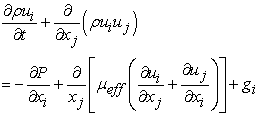(3)

where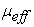is the effective viscosity defined as: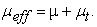(4)

Two-equation k-ε model is used to model turbulence flow, in which k represents turbulent kinetic energy of flow, and ε is dissipation rate of energy. In this model, eddy viscosity (5) is related to viscous kinetic energy and dissipation rate: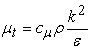(5)

where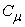is constant and k, ε are determined by solving the following transport equations: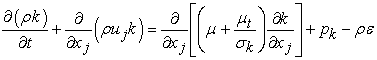(6)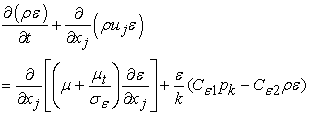(7)

where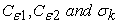are constant values, and Pk is turbulence generation due to viscous forces. The VOF model is used to simulate complex deformations at the interface of air-water. In this case, the following transport equation is solved to calculate volume fraction of water to air in each time step.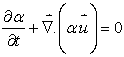(8)

where: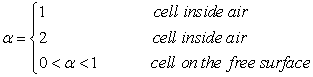In this model, the effective density and viscosity in each computational cell are used to solve the Navier-Stokes equations, which are calculated from the following equations: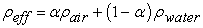(9)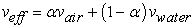(10)

where the subscripts 1 and 2 represent water and air respectively.

The propeller's hydrodynamic characteristics can be defined using advance coefficient, thrust coefficient, torque coefficient, and efficiency. When the propeller is placed in front of the oblique flow at various angles the advance coefficient, thrust coefficient, torque coefficient, and efficiency are defined in three coordinate directions using the following equations: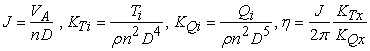(11)
2.2. Computational Domain and Mesh

The flow around the propeller is complex because of its geometry so, to perform accurate simulation must use appropriate computational domain. Two cylinders are used to simulate the flow around the propeller. The inlet flow in the upstream is located at distance 3D and the outlet flow in downstream, is located at distance 7D from the propeller. This problem is controlled by boundary conditions of the wall, velocity inlet, pressure outlet that applied to the solution domain Figure 1. The normal velocity magnitude in the velocity inlet boundary condition is defined according to the rotational velocity which its measure is 25 RPS. In this paper non-slip condition is used for walls. The non-slip condition on the propeller surface allows for the calculation of lift and drag forces because in the propeller this is a lift force that generates thrust. As well as the boundary condition for the volume control is symmetric because a stream is simulated with an infinite boundary and far from the propeller. In present study, structural meshing is used and else to verify the sensitivity of the mesh, the thrust coefficient is compared in numerical and experimental methods. In this section, three types of meshing are used including small, medium and large and the percentage error obtained against each one. The mesh sensitivity is shown in Table 1. Since the accuracy of the small grid is very high it is used for meshing of volume control and also this method of meshing is used for other modes, as shown in Figure 2.

• Figure 1. Computational domain
• Figure 2. Meshing of the domain and propeller

### 3. Results and Discussions

The hydrodynamic characteristics numerical results of the propeller are evaluated at various operating conditions. Two types of simulations are conducted in this study. First, the propeller is examined in direct flow and without angle and compared with experimental results and then simulation of the flow is done for four different angles. This part of the research is conducted within the range of the advance coefficient of 0.1 to 0.8. In order to verify the simulation done on the propeller the results are compared with experimental results to examine simulation accuracy. Figure 3 shows the comparison of the open-water characteristics of the numerical and experimental data. As it is observed the numerical results have in good agreement with experimental data.

So after verifying the results other simulations with different angles of current carried out within the same range of advance coefficient. As it mentioned the angles of the collision current to the propeller are 10, 20, 30 and 40 degrees so at each step of the analysis, the angle of current is added 10 degrees. Figures 4 and 6 are presented the thrust and torque coefficients again flow angle at four advance velocity coefficients. When the angle increases the both thrust and torque coefficients are diminished. The thrust coefficient reduction in the advance coefficient of 0.1, 0.29, 0.5 and 0.67 is 21.8%, 17.4%, 21.1% and 22.9% respectively. The reason for reducing the thrust coefficient in this study is the angle of the current in contact with the propeller and the direction of propeller rotation. Lateral force coefficient and torque coefficient against flow angle in various advance coefficients are shown in Figure 5 and Figure 7. When the flow angles increase the lateral force coefficients are linearly increased at all advance coefficients. But the torque coefficients are linearly increased at low advance coefficients (J=0.1, 0.29) and nonlinearly at high coefficients (J0.5, 0.67).

• Figure 3. Comparison of the numerical results and experimental data

The velocity contour around the propeller is shown at the advance coefficient of 0.29 and at the four obliges angles of flow shown in Figure 8 and Figure 9 at various angle flows. The angle 10 and 20 degrees, it is shown in Figure 8 and the angles of 30 and 40 degrees are shown in Figure 9. In addition, pressure contour on the propeller surface in the advance coefficient of 0.5 and at the angle of flow (30 deg) shown in Figure 10. The pressure contours at both face and back sides are presented.

• Figure 4. Thrust coefficient against flow angle
• Figure 5. Lateral force coefficient against flow angle
• Figure 6. Torque coefficient against flow angle
• Figure 7. Torque coefficient around the Y-axis
• Figure 8. Velocity contour around the propeller at J=0.29 and flow angle of 100 and 200
• Figure 9. Velocity contour around the propeller at J=0.29 and flow angle of 300 and 400
• Figure 10. Pressure contour on the propeller surface at J=0.5 and flow angle of 300

### 4. Conclusion

The article is presented the hydrodynamic characteristics of the propeller (B:4-70) by using CFD StarCCM+ software. The open-water characteristics of the propeller are calculated and compared with experimental data. Then, many numerical results like thrust and torque coefficients lateral force at various oblique angles, velocity contour and pressure contour are presented and discussed.

### References

  Shamsi, R., Ghassemi, H., Determining the hydrodynamic loads of the marine propeller forces in oblique flow and off-design condition. Iran. J. Sci. Technol. Trans. Mech. Eng. 41, 2017, pp121-127. In article View Article  Shamsi, R., Ghassemi, H., Numerical investigation on yaw angle effects on propulsive characteristics of podded propulsions. Int. J. Nav. Archit. Ocean Eng. 5, 2013, 287-301. In article View Article  Shamsi R., Ghassemi H., Iranmanesh M., A Comparison of the BEM and RANS Calculations for the hydrodynamic performance of the PODS, Mechanics & Industry 18, 205, 2017. In article View Article  Viviani M, Podenzana Bonvino C, Mauro S, Cerruti M, Guadalupi D, Menna A., Analysis of asymmetrical shaft power increase during tight manoeuvre. In: 9th Int. conf. on high performance marine vehicles, Shangai, China, 2007. In article  Chang, P., Elbert, M., Young, Y., Liu, Z., Mahesh, K., Jang, H., Propeller forces and structural response due to crashback. In: Proc. of 27th Symp. on Naval Hydrodynamic, Seoul, Korea, 2008. In article  Atsavapranee P, Miller R, Day C, Klamo J, Fry D., Steady-turning experiments and RANS simulations on a surface combatant hull form (Model#5617). In: Proc. of 28th Symp. on naval hydrodynamics, Pasadena, California, 2010. In article  Dubbioso, G., Muscari, R., Mascio, A.D., Analysis of the performance of a marine propeller operating in oblique flow, Comput. Fluid 75, 2013, 86-102. In article View Article  Dubbioso, G., Muscari, R., Mascio, A.D., Analysis of a marine propeller operating in oblique flow. Part 2: very high incidence angles. Comput. Fluid 92, 2014, 56-81. In article View Article  Shuai, R., Liang, L., Chao, W., Hongyu, Z., Numerical prediction analysis of propeller exciting force for hull-propeller-rudder system in oblique flow. Int. J. Nav. Archit. Ocean Eng. 10, 2018, 69-84. In article View Article  Wang, Chao, Sun, Shuai, Li, Liang, Ye, Liyu, Numerical prediction analysis of propeller bearing force for full-scale hull-propeller-rudder system. Int. J. Nav. Archit. Ocean Eng. 8, 2016, 589-601. In article View Article  Nowruzi, H., Ghassemi, H. and Ghiasi, M., 2017. Performance predicting of 2D and 3D submerged hydrofoils using CFD and ANNs. Journal of Marine Science and Technology, 22(4), pp.710-733. In article View Article  Shora, M.M., Ghassemi, H. and Nowruzi, H., 2017. Using computational fluid dynamic and artificial neural networks to predict the performance and cavitation volume of a propeller under different geometrical and physical characteristics. Journal of Marine Engineering & Technology, pp.1-26. In article  Najafi, A., Nowruzi, H. and Ghassemi, H., 2018. Performance prediction of hydrofoil-supported catamarans using experiment and ANNs. Applied Ocean Research, 75, pp.66-84. In article View ArticleThis work is licensed under a Creative Commons Attribution 4.0 International License. To view a copy of this license, visit http://creativecommons.org/licenses/by/4.0/